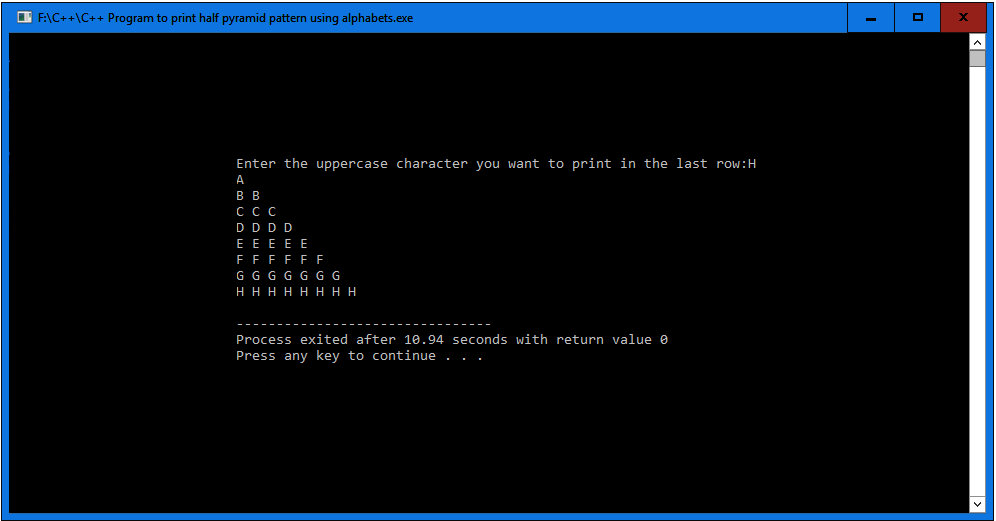# C++ Program to print half pyramid pattern using alphabets

In this article write a C++ Program to print half pyramid pattern using alphabets. This Program first takes the numbers of rows and uses nested for loops to print half pyramid pattern.

## C++ Program to print half pyramid pattern using alphabets

```// C++ Program to print half pyramid pattern using alphabets
#include <iostream>
using namespace std;

int main()
{
char input, alphabet = 'A';
int i, j;

cout << "Enter the uppercase character you want to print in the last row:";
cin >> input;

// outer loop is responsible for rows
for(int i = 1; i <= (input-'A'+1); i++)
{

//inner loop is responsible for columns
for(int j = 1; j <= i; j++)
{
cout << alphabet << " ";
}
alphabet++;

// give line breaks after ending every row
cout << "\n";
}
return 0;
}
```

### When the above C++ program is compile and run, this will produce the following result: